# Step-by-step Solutions for Definite Integrals in Wolfram|Alpha

November 11, 2014 —Comments Off

One of the most popular queries on Wolfram|Alpha is for definite integrals. So we’re especially excited to announce that Step-by-step solutions for these are now available! The general method used to find the steps for definite integrals is to tap into the already existing “Show steps” functionality for indefinite integration, and then to use the fundamental theorem of calculus.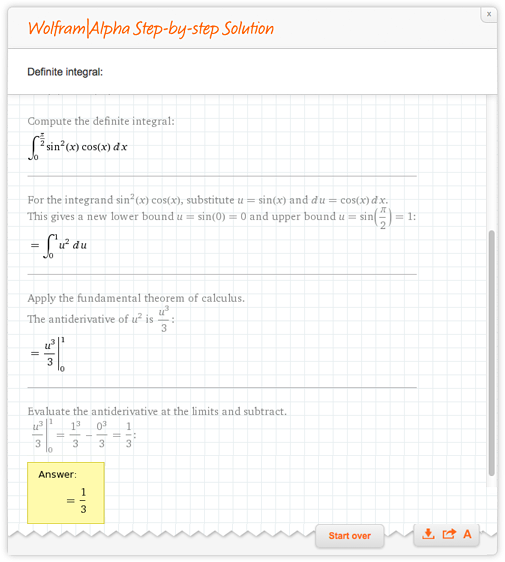Now you may think it was trivial to add this functionality given that indefinite integrals already have steps, but there are many tricky cases to consider: before we even begin to integrate, the continuity of the function is examined. If there are discontinuities in the integration domain, the domain is split and the integral is evaluated separately over each domain.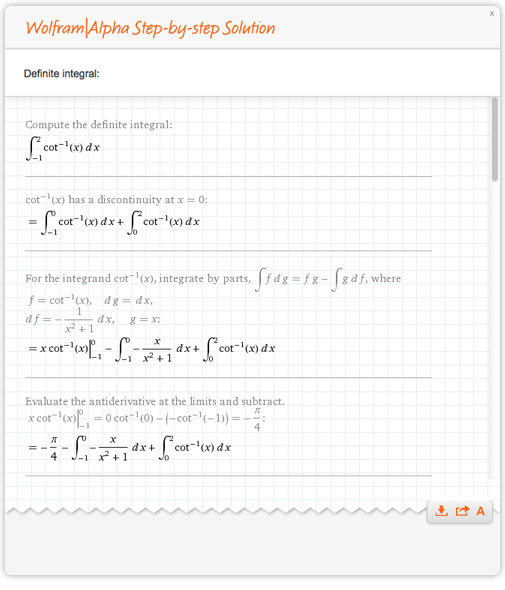We must determine if the integral is proper or improper.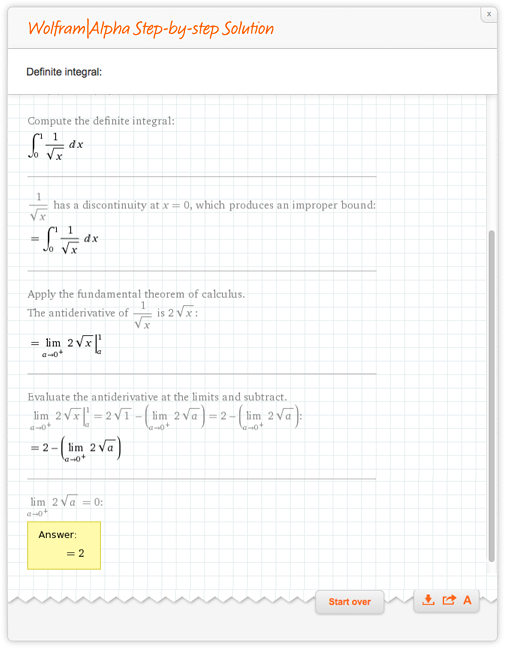Absolute values need to be handled carefully.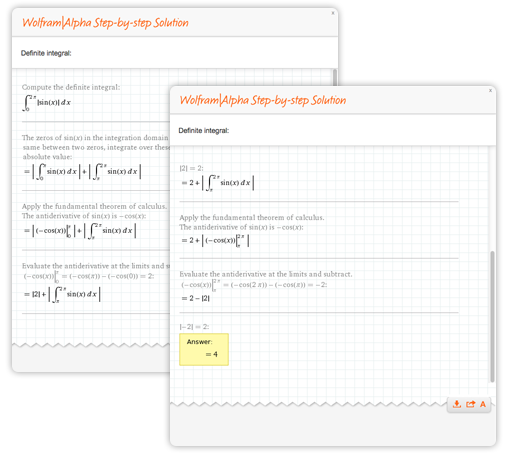Symmetries can be exploited.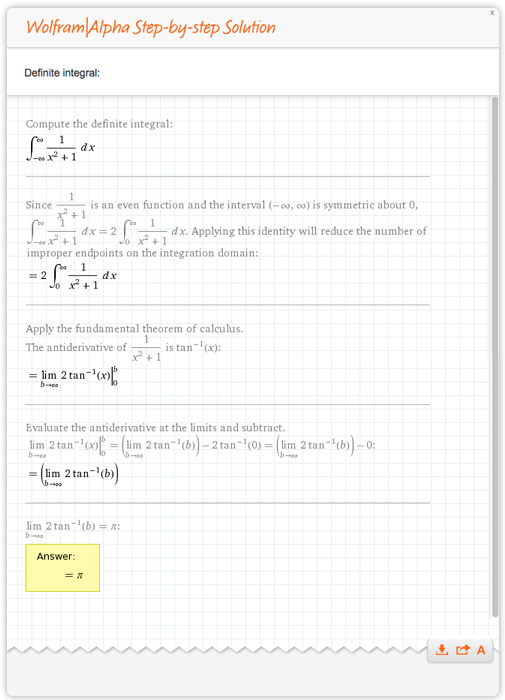Simplification of radicals and logarithms must be done very carefully.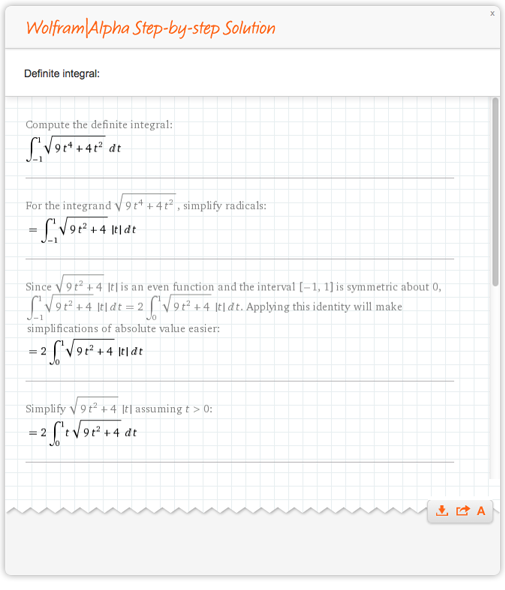Finally, the fundamental theorem of calculus requires that the antiderivative is continuous over the integration domain (see this blog post for more information). Therefore, we need to be careful when finding the indefinite integral, and always ensure the result will be continuous. One way to do this is to detect when we will have a discontinuous antiderivative and split the integration domain up.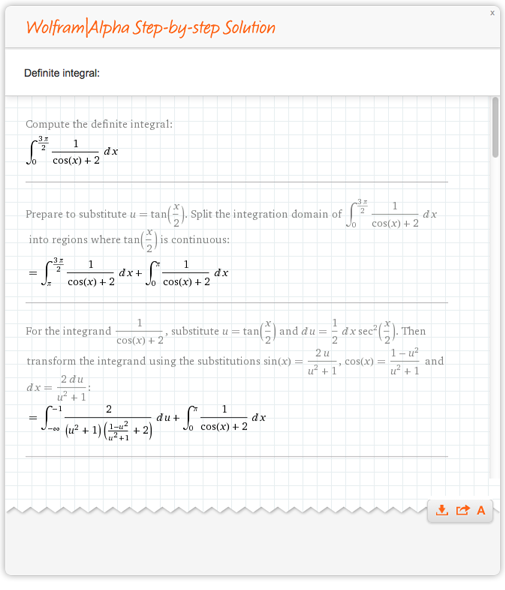Integration is an extremely nontrivial problem, so we hope these Step-by-step solutions will help you learn how they can be done. Be sure to check out Step-by-step solutions for other topics too.

I’m curious, on a semi-related note I just ran an integral through using “integrate cos(e^t) on [x,y]” and I don’t get why mathmatica returns that obscure Ci(x) function why not just keep it in terms of sin?

Posted by Ian November 14, 2014 at 12:36 am

Thank you for your comment, this function cos(e^t) does not have an elementary antiderivative. To see this, substitute u = e^t, du = e^tdt, i.e. dt = du/u. This means

int cos(e^t) dt = int cos(u)/u du = Ci(u) + c = Ci(e^t) + c,

where the second equality is by definition. See http://mathworld.wolfram.com/CosineIntegral.html for more information on Ci(x).

Posted by The Wolfram Team November 17, 2014 at 11:51 am

Hi Greg,
Wonderful piece of information on step by step feature. Is there a post that talks about the generated plots of the integrals and the interactivity feature which is not available to general users?

Thanks,
Amit

Posted by Amit December 15, 2014 at 7:34 am

Thank you for comment, at this time we do not have a post that fits the description you inquired about.

Posted by The Wolfram Team December 16, 2014 at 10:40 am

Posted by Mamad February 5, 2015 at 12:03 pm

Step by step solution is very useful feature. Thank you!

Posted by Mat Ma February 23, 2015 at 3:57 pm

I have a problem in integrating this expression

(sin(x)^2)/(1+k*cos(x))^3

I did the indefinite integral using Wolfran Alpha and then I use the resulting expression to calculate the value of the function over an interval. However, I solve the same integral, but this time I calculated the define integral over the same interval I did before. It surprises me that the results were different calculating in both ways. Do you have any idea about what’s is going on?

PS: I did the numerical integral using my own code and the result is equal to the one obtained using the definite integral.

Thanks a lot for your help

Posted by Tiago March 27, 2015 at 7:53 pm

You have not provided a step by step solution to my question before, which is the integral
of xsinx/(x^2 + 4x + 5 ) form – infinity to + infinity. Please tell me how I may find this solution, since I cannot find it in any computer site or textbook. I am trying to solve it via contour integration.